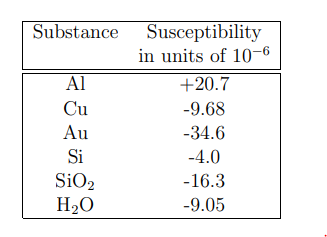Skip to main content
$$\require{cancel}$$

# 6.1: Introduction to Ferromagnetism

The magnetization density, $$\vec M$$, in most materials at room temperatures is proportional to the magnetic field, $$\vec H$$:

$\vec{\text{M}}=\chi \vec{\text{H}} , \label{6.1}$

The factor of proportionality, $$\chi$$, is called the magnetic susceptibility. Since $$\vec M$$, and $$\vec H$$ have the same units (Amps/meter) the magnetic susceptibility has no dimensions. Typical values of the susceptibility at room temperatures for some common substances are listed in Table (6.1.1). It is clear from this Table that for such non-magnetic substances the magnetization per unit volume is negligible compared with values of the impressed magnetic field, $$\vec H$$. The situation is quite different for ferromagnetic substances such as iron, nickel, or cobalt. In a ferromagnet the magnetic moments are held parallel by very strong forces called exchange forces. The magnetization per unit volume, $$| \vec M_s |$$, is very large and essentially independent of applied magnetic field at temperatures low compared with a critical temperature called the Curie Temperature. The Curie temperature, Tc, is that temperature at which the magnetization in zero applied magnetic field goes to zero. The Curie temperature is material dependent. The Curie temperatures for iron,nickel and cobalt are 1044, 631, and 1393 K respectively. The magnetization varies slowly with temperature at low temperatures. The temperature dependence of the magnetization in iron is depicted in Figure (6.1.1). The temperature dependence of Ms for other ferromagnets is very similar. If a cylindrical rod of iron were to be uniformly magnetized along its length the magnetic field strength near its end surfaces would be very large, see Equation (4.3.12) and Figure (4.3.11) of Chapter(4).Table $$\PageIndex{1}$$: The magnetic susceptibility at room temperature for some common non-magnetic substances.Figure $$\PageIndex{1}$$: The variation with temperature of the reduced magnetization for pure iron. The Curie temperature is Tc = 1044 K, and the magnetization at T=0 K is Ms(0) = 22.1 kOe= 1.76 × 107 Amps/m.

The field just outside an end face and near the cylinder axis is given by Bz = µ0M0/2 for a cylinder whose length, Ld, is much greater than its radius, R. For iron at room temperature this field is approximately 1 Tesla. It is, however, common experience that the fields around a length of iron rod are very weak, of the order of 0.01 Teslas or less. The field outside the rod is weak because the magnetization is broken up into a very large number of small domains. Each domain carries a large magnetization, Ms , but the direction of the magnetization changes from domain to domain in such a way that the average magnetization density is very nearly zero. It can be shown that the energy due to a magnetization distribution can be calculated from

$\text{U}_{\text{m}}=\frac{\mu_{0}}{2} \int \int \int_{S p a c e} \text{d} \tau \text{H}_{\text{m}}^{2}, \label{6.2}$

where $$H_m$$ is the magnetic field generated by the magnetic charge density $$\rho_{m}=-\operatorname{div}(\vec{\text{M}})$$ and d$$\tau$$ is the element of volume. It follows that in the absence of an applied external field the domain magnetization vectors will attempt to orient their magnetization vectors so as to make (\ div (\vec M\)) as nearly zero as possible. This tendency is called ”the magnetic pole avoidance principle”. The size of the magnetic domains in the absence of an applied magnetic field depends very strongly on the structure of the material (whether the specimen is a polycrystal or a single crystal), upon the concentration of impurities, and upon the presence of internal stresses. The domain dimensions in an annealed polycrystalline iron bar are of the order of 1/10 mm on a side. The domains are therefore very large compared with atomic dimensions, but are small on a macroscopic scale. In very perfect single crystalline prisms of iron in which the cubic iron axes are accurately parallel with the edges of the specimen the domains may be as long as a cm or more: see Figure (6.1.2).Figure $$\PageIndex{2}$$: The domain structure at room temperature in a perfect iron single crystal in which the edges of the crystal are accurately parallel with the crystalline axes. The magnetization is uniform along the z-direction (out of the paper). Simple domain structures are observed only in single crystalline specimens.
• Was this article helpful?